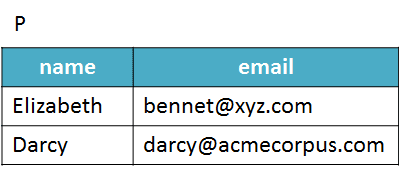# Set Operations Applied to Pandas DataFrames

In this tutorial, we show how to apply mathematical set operations (union, intersection, and difference) to Pandas DataFrames with the goal of easing the task of comparing the rows of two datasets.

By Eduardo Corrêa Gonçalves, ENCE/IBGE

### Introduction

In certain practical situations, it might be interesting to treat a pandas DataFrame as a mathematical set. In this case, each row of the DataFrame can be considered as an element or member of the set.

The question then becomes: Why would it be useful? Here’s the answer. As we know, data science problems typically require the analysis of data obtained from multiple sources. At some point in the analysis of data from a study, you may face the problem of having to compare the contents of two or more DataFrames to determine if they have elements (rows) in common. In this tutorial you will learn that set operations are one of the best and most natural techniques you can choose to perform such a task.

### A Practical Example

Suppose you have two DataFrames, named P and S, which respectively contain the names and emails from students enrolled in two different courses, SQL and Python.Consider that you need answers to the following questions:

1. How many different students are in the two DataFrames?
2. Are there students enrolled in both courses, Python and SQL?
3. Which students are taking the Python course, but not the SQL course (and vice versa)?

Answers can be obtained in a straightforward way if you treat the DataFrames as two distinct mathematical sets. Then, all you will have to do is to apply the basic union, intersection, and difference set operations:

P ∪ S, the union of P and S, is the set of elements that are in P or S or both. Note that the element (student) Elizabeth appears only once in the result.P ∩ S, the intersection of P and S, is the set of elements that are in both P and S. Now, only Elizabeth appears, because she is the only in both sets.P − S, the difference of P and S, is the set that includes all elements that are in P but not in S:Note that S − P is different from P − S:It is important to remark that the DataFrames on which any of these three operations are applied must have identical attributes (as shown in the example).

### Set Operations in Pandas

Although pandas does not offer specific methods for performing set operations, we can easily mimic them using the below methods:

• Union: concat() + drop_duplicates()
• Intersection: merge()
• Difference: isin() + Boolean indexing

In the following program, we demonstrate how to do it. A detailed explanation is given after the code listing.

Results are shown below:

```------------------------------
all students (UNION):
name                 email
0  Elizabeth        bennet@xyz.com
1      Darcy  darcy@acmecorpus.com
2    Bingley       bingley@xyz.com
------------------------------
Students enrolled in both courses (INTERSECTION):
name           email
0  Elizabeth  bennet@xyz.com
------------------------------
Python students who are not taking SQL (DIFFERENCE):
name                 email
1  Darcy  darcy@acmecorpus.com
------------------------------
SQL students who are not taking Python (DIFFERENCE):
name            email
0  Bingley  bingley@xyz.com```

Here's the complete explanation of the code. Initially, we created two DataFrames, P (Python students) and S (SQL students). Once created, they were submitted the three set operations in the second part of the program.

### Union

To perform the union operation, we applied two methods: concat() followed by drop_duplicates(). The first accomplishes the concatenation of data, which means to place the rows from one DataFrame below the rows of another DataFrame. Thus, the following statement:

`all_students = pd.concat([P, S], ignore_index = True) `

generates a DataFrame composed of 4 rows (2 rows from P plus 2 from S).

```        name                 email
0  Elizabeth        bennet@xyz.com
1      Darcy  darcy@acmecorpus.com
2    Bingley       bingley@xyz.com
3  Elizabeth        bennet@xyz.com```

However, note that there are two rows referring to Elizabeth, since she is the only student who is enrolled in both courses. To keep only one occurrence of this element it is enough to use the drop_duplicates() method:

`all_students = all_students.drop_duplicates() `

```        name                 email
0  Elizabeth        bennet@xyz.com
1      Darcy  darcy@acmecorpus.com
2    Bingley       bingley@xyz.com```

### Intersection

The versatile merge() method was employed to execute the intersection operation. This method can be used to combine or join DataFrames in different ways. However, when used without the specification of any parameter in an operation involving two compatible DataFrames, it yields their intersection:

`sql_and_python = P.merge(S)`

```        name           email
0  Elizabeth  bennet@xyz.com```

### Difference

The difference operation has a slightly more complicated code. As we know, the difference between two sets P and S is the operation that aims to determine the elements of P that are not part of S. In pandas, we can implement this operation using the isin() method in tandem with boolean indexing:

`python_only = P[P.email.isin(S.email) == False]`

To explain this statement, we will break it into two parts. The first is:

`P.email.isin(S.email)`

The above command produces a boolean structure that points out which emails in the DataFrame P are contained in S:

```0     True
1    False```

This boolean structure is then used to filter rows from P:

`python_only = P[P.email.isin(S.email) == False]`

```    name                 email
1  Darcy  darcy@acmecorpus.com```

Obtaining the SQL students who are not taking Python is done analogously:

`sql_only = S[S.email.isin(P.email) == False]`

```      name            email
0  Bingley  bingley@xyz.com```

Pandas documentation
https://pandas.pydata.org/pandas-docs/stable/

Stanford Encyclopedia of Philosophy - Basic Set Theory
https://plato.stanford.edu/entries/set-theory/basic-set-theory.html

Jenifer Widom - Relational algebra 2 part 1